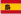DAWSON GROUP LTD.Español
Estás aquí: » » » » » RBS28A BS 1500KG / 3300LBS Hebilla de trinquete de acero inoxidable AISI 304Compartir con:

# RBS28A BS 1500KG / 3300LBS Hebilla de trinquete de acero inoxidable AISI 304

Modelos de venta: mayorista
Pedido mínimo: 1000pcs
• DAWSON/OEM

• RBS28A

• 1 1/16 "

• 1000pcs

### RBS28A BS 1500KG / 3300LBS Hebilla de trinquete de acero inoxidable AISI 304

- Dawson Group Ltd. - Fabricante, proveedor, fábrica de ChinaRBS28A

A = 28, B = 40, C = 128

BS: 1500 KG / 3300 LBS
Show de productos:RBS50C   A = 54, B = 60, C = 230   BS: 3,000KG / 6,600LBSRBS50M   A = 54, B = 60, C = 192   BS: 3,000KG / 6,600LBSRBS50T   A = 54, B = 60, C = 166   BS: 3,000KG / 6,600LBSRBS50X   A = 52, B = 43, C = 128   BS: 2,000KG / 4,400LBSRBS38A   A = 41, B = 60, C = 166   BS: 2,500 KG / 5,500LBSRBS38B   A = 41, B = 41, C = 127   BS: 2,000 KG / 4,500 LBSRBS28A   A = 28, B = 40, C = 128   BS: 1,500 KG / 3,300 LBSRBS28B   A = 28, B = 40, C = 119   BS: 1,500 KG / 3,300 LBSRBS25   A = 27, B = 40, C = 115   BS: 800 KG / 1,760 LBSA: ancho de banda (mm)   B: Espesor (mm)   C: Longitud del brazo (mm)OBS5004   A = 28, B = 40, C = 128   BS: 1,500 KG / 3,300 LBS### RBS28A BS 1500KG / 3300LBS Hebilla de trinquete de acero inoxidable AISI 304

- Dawson Group Ltd. - Fabricante, proveedor, fábrica de ChinaRBS28A

A = 28, B = 40, C = 128

BS: 1500 KG / 3300 LBS
Show de productos:RBS50C   A = 54, B = 60, C = 230   BS: 3,000KG / 6,600LBSRBS50M   A = 54, B = 60, C = 192   BS: 3,000KG / 6,600LBSRBS50T   A = 54, B = 60, C = 166   BS: 3,000KG / 6,600LBSRBS50X   A = 52, B = 43, C = 128   BS: 2,000KG / 4,400LBSRBS38A   A = 41, B = 60, C = 166   BS: 2,500 KG / 5,500LBSRBS38B   A = 41, B = 41, C = 127   BS: 2,000 KG / 4,500 LBSRBS28A   A = 28, B = 40, C = 128   BS: 1,500 KG / 3,300 LBSRBS28B   A = 28, B = 40, C = 119   BS: 1,500 KG / 3,300 LBSRBS25   A = 27, B = 40, C = 115   BS: 800 KG / 1,760 LBSA: ancho de banda (mm)   B: Espesor (mm)   C: Longitud del brazo (mm)OBS5004   A = 28, B = 40, C = 128   BS: 1,500 KG / 3,300 LBSAnterior:
Siguiente:
>Si tiene alguna sugerencia u opinión sobre nuestros productos, deje un mensaje y responderemos sus preguntas de inmediato.
Peticiones sobre producto